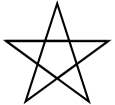# Chapter 5 Exercises¶

1. There are 3 syntax errors in the following code. Fix it to work correctly without errors.

Change `screen` to `Screen`. Change `Turtle` to `Turtle()`. Change `Forward` to `forward`.

2. The code below is correct, but the lines are in the wrong order. Fix it so that it runs properly.

The import statement has to come first and then you have to define the screen and then the turtle before you can draw.

3. The following program is missing things on lines 1, 2, and 3. Add the missing parts.

Finish the import. Create the space using `Screen()`. Create the turtle using `Turtle()`.

4. Rearrange the code so it draws a square.

For a square, it needs to go forward and then turn 90 until the square is complete.

5. The following code has 3 syntax errors. Fix the errors so that the code runs.

Change `turtle()` to `Turtle()`. Change `Forward` to `forward`. Change `turn` to `left`.

6. Fix the 6 errors in the following code.

Add the `*` on line 1 to import all modules from the turtle module. `Screen` is a method so it must have `()`. On line 3, `Turtle()` needs to be capitalized. On lines 4,5, and 8, `forward()` and `left()` should be lowercase.

7. The following code draws two lines of a rectangle. Add code to finish drawing the rectangle.

Add another `alex.left(90)` and then copy and paste lines 4-6 to the end.

8. You need to fix or add 4 things so that the code runs.

You have to import the turtle module first. On line 3, red should be a string. On line 4 `forward()` should be lowercase and 50 should be an int.

9. The following code is missing 3 lines that do the required set-up. Add them so that the code runs.

You must import the turtle library, create a drawing space, and create the turtle.

10. Finish the code so that it draws an equilateral triangle.

You have to turn the turtle 120 degrees left and then go forward 150 units twice.

11. Create a drawing that includes penup, pendown, and pensize.

Here is one example.

12. Fix the 5 errors.

On line 1, from and import should be lowercase. On line 2, `Screen()` should be uppercase. On line 3, it should be `Turtle()`. For the last error, you can either change `bob` on line 3 or line 4, but they should be the same.

13. Create a drawing with at least 3 colors and using at least 3 turtles.

Here is one example.

14. Fix the errors.

The correct method is `pensize()` and you can only call the methods on jill because that is the name of the turtle.

15. Write code below to draw a diamond shape.

Here is one example.

16. Write code that spells CS in block letters (it will look more like C5).

17. Write code below to draw a star like this picture.Here is one example.